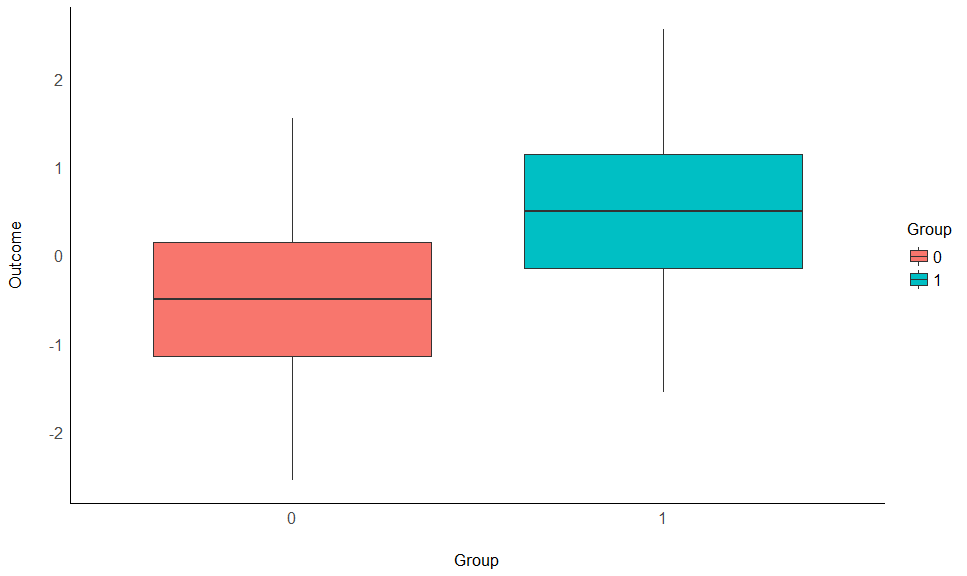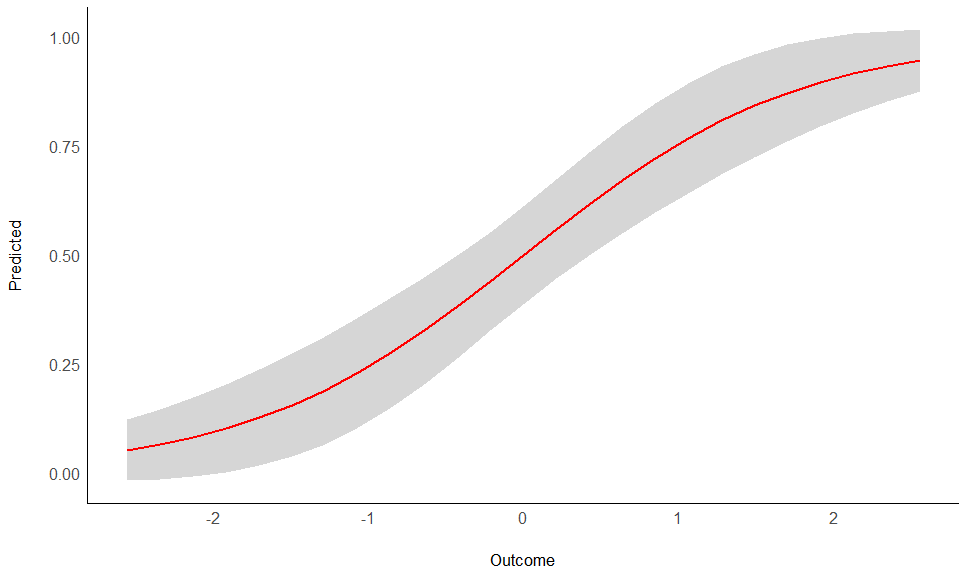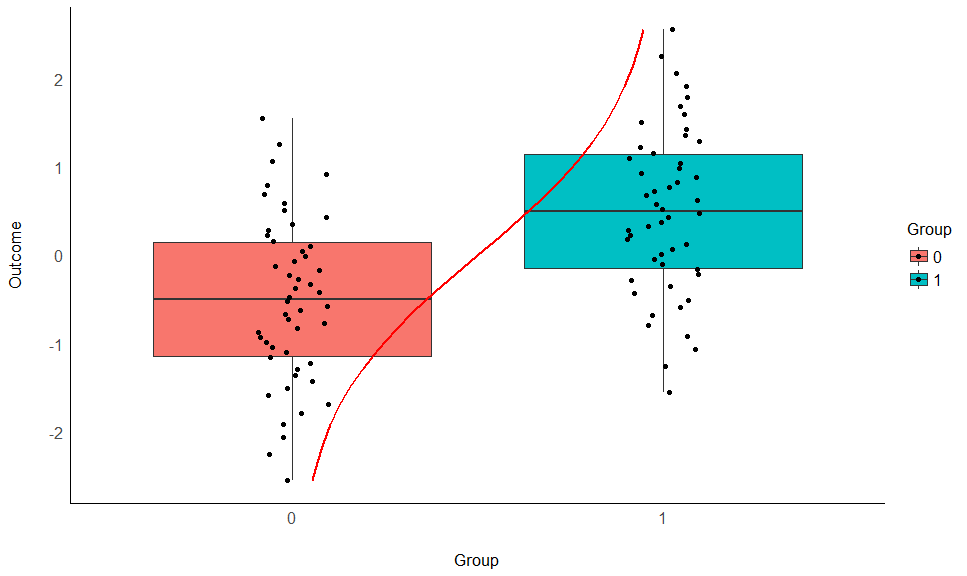# Odds Ratio

This vignette needs some love.

# Validate a t-test with Logistic Regression

Let’s start off with a simple example. We will simulate 100 observations of a normally distributed outcome variable (mean = 0 and SD = 1) and a grouping variable made of zeros and ones. Importantly, the mean difference between these two groups (zeros vs. ones) is of 1.

data <- bayestestR::simulate_difference(n = 100,
d = 1,
names = c("Group", "Outcome"))

summary(data)
>  Group     Outcome
>  0:50   Min.   :-2.55
>  1:50   1st Qu.:-0.74
>         Median : 0.00
>         Mean   : 0.00
>         3rd Qu.: 0.74
>         Max.   : 2.55


Now, as we are interested in the difference between these two groups, we can first investigate it using a t-test.

library(ggplot2)

ggplot(data, aes(x=Group, y=Outcome, fill=Group)) +
geom_boxplot() +
see::theme_modern()ttest <- t.test(Outcome ~ Group, data=data, var.equal=TRUE)
ttest_pars <- parameters::parameters(ttest)
ttest_pars
> Parameter | Group | Mean_Group1 | Mean_Group2 | Difference |     t | df |      p |         95% CI |             Method
> ----------------------------------------------------------------------------------------------------------------------
> Outcome   | Group |       -0.50 |        0.50 |       1.00 | -5.32 | 98 | < .001 | [-1.37, -0.63] |  Two Sample t-test


As we can see, this confirms our simulation specifications, the difference is indeed of 1.

## Cohen’s d

Let’s compute now, a traditional Cohen’s d using the effectsize package. While this d should be close to 1, it should theoretically be a tiny bit larger, because it takes into account the (pooled) SD of the whole variable x (across the groups), which because of the difference is a bit larger than 1.

sd(data$Outcome) >  1.1  We can compute the Cohen’s d as follows: effectsize::cohens_d(data$Outcome, data$Group) > Cohen's d | 95% CI > -------------------------- > -1.06 | [-1.48, -0.64]  As expected, it’s pretty close to 1 times the SD of the sample. Interestingly, one can estimate the Cohen’s d directly from the result of the t-test, using the t statistic. We can convert it to a d using the effectsize package: effectsize::t_to_d(ttest_pars$t, df_error = ttest_pars\$df)
>     d |         95% CI
> ----------------------
> -1.07 | [-1.50, -0.65]


Fortunately, they are quite close.

## The Complimentry Logistic Model

Another way of investigating these differences is through the lens of a logistic regression. The main difference is that here, the group variable y becomes the outcome and x the predictor. Let’s fit such model and investigate the parameters:

model <- glm(Group ~ Outcome, data = data,
family = "binomial")

parameters::parameters(model)
> Parameter   | Coefficient |   SE |        95% CI |        z |      p
> --------------------------------------------------------------------
> (Intercept) |    1.23e-16 | 0.23 | [-0.45, 0.45] | 5.41e-16 | > .999
> Outcome     |        1.13 | 0.27 | [ 0.65, 1.70] |     4.25 | < .001


How to interpret this output? The coefficients of a logistic model are expressed in log-odds, which is a metric of probability. Using the modelbased package, one can easily visualize this model:

data_grid <- modelbased::estimate_link(model)

ggplot(data_grid, aes(x = Outcome, y = Predicted)) +
geom_ribbon(aes(ymin = CI_low, ymax = CI_high), alpha = 0.2) +
geom_line(color = "red", size = 1) +
see::theme_modern()We can see that the probability of y being 1 (vs. 0) increases as x increases. This is another way of saying that there is a difference of x between the two groups of y. We can visualize all of our this together as follows:

ggplot(data, aes(x=Group, y=Outcome, fill=Group)) +
geom_boxplot() +
geom_jitter(width = 0.1) +
# add vertical regression line
geom_line(data = data_grid,
aes(x = Predicted + 1, y = Outcome, fill = NA),
color = "red", size = 1) +
see::theme_modern()You can notice that the red predicted probability line passes through x=0 when y=0.5. This means that when x=0, the probability of the two groups is equal: it is the “middle” of the difference between them.